maths > mensuration-high

Measurement by superimposition

what you'll learn...

Overview

Measurement by Superimposition : Length, Area, or Volume can be measured by superimposition of corresponding unit-measures.overlay a grid

Consider the shape given in the figure. It is a rectangle of length $7$$7$ cm and width $5$$5$ cm.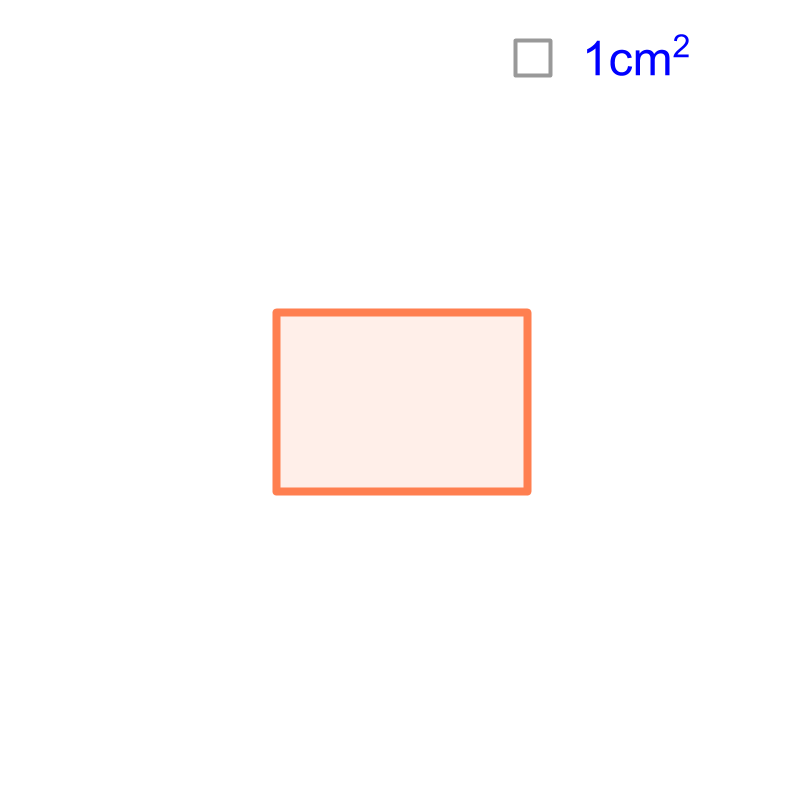The figure also provides the reference unit-square on the top-right. The area of the shape is "$35c{m}^{2}$$35 c {m}^{2}$". The area is computed by creating a grid of unit-squares. The number of unit squares contained within the shape is the area of the rectangle.simplify

Area of the given shape is calculated by superimposing the reference unit-squares. Based on this, the area of a square or rectangle is simplified to the formulas

$\text{Area of a square}\phantom{\rule{1ex}{0ex}}={\phantom{\rule{1ex}{0ex}}\text{side}}^{2}$$\textrm{A r e a o f a \square} = {\textrm{s i \mathrm{de}}}^{2}$

$\text{Area of a rectangle}\phantom{\rule{1ex}{0ex}}=\phantom{\rule{1ex}{0ex}}\text{length}\phantom{\rule{1ex}{0ex}}×\phantom{\rule{1ex}{0ex}}\text{width}$$\textrm{A r e a o f a r e c \tan g \le} = \textrm{\le n > h} \times \textrm{w i \mathrm{dt} h}$

approximate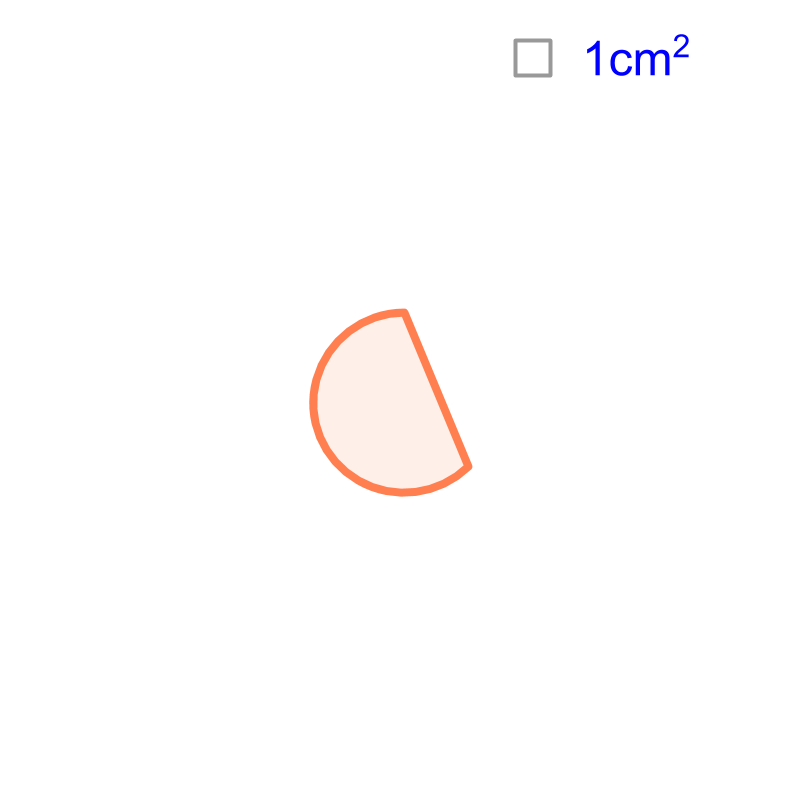Consider the shape given in the figure. The measurements are not provided. The reference unit-square is shown on the top-right corner. Can we calculate the area of the given shape? Yes, "approximate area can be calculated by superimposing a grid of unit-squares".

The area is approximately $15c{m}^{2}$$15 c {m}^{2}$.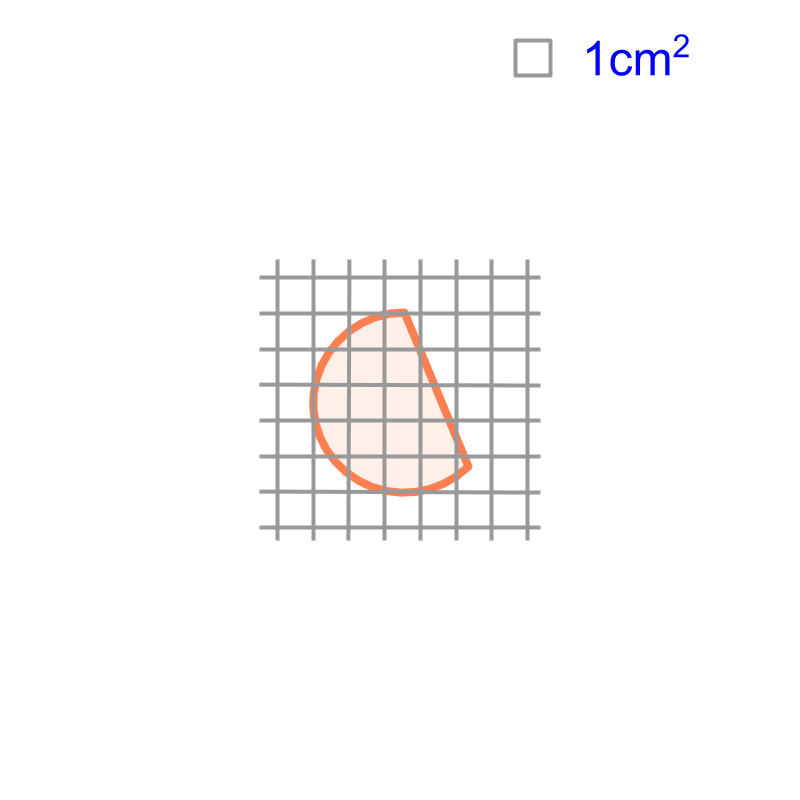.
The area is approximated to the count of large squares within the figure.

suitable for some shapes

Measurement by superimposition provides accurate results for

•  area of squares and rectangles : The unit-square has ${90}^{\circ }$${90}^{\circ}$ angles at the vertices. And so, the unit-square fits within Squares and Rectangles.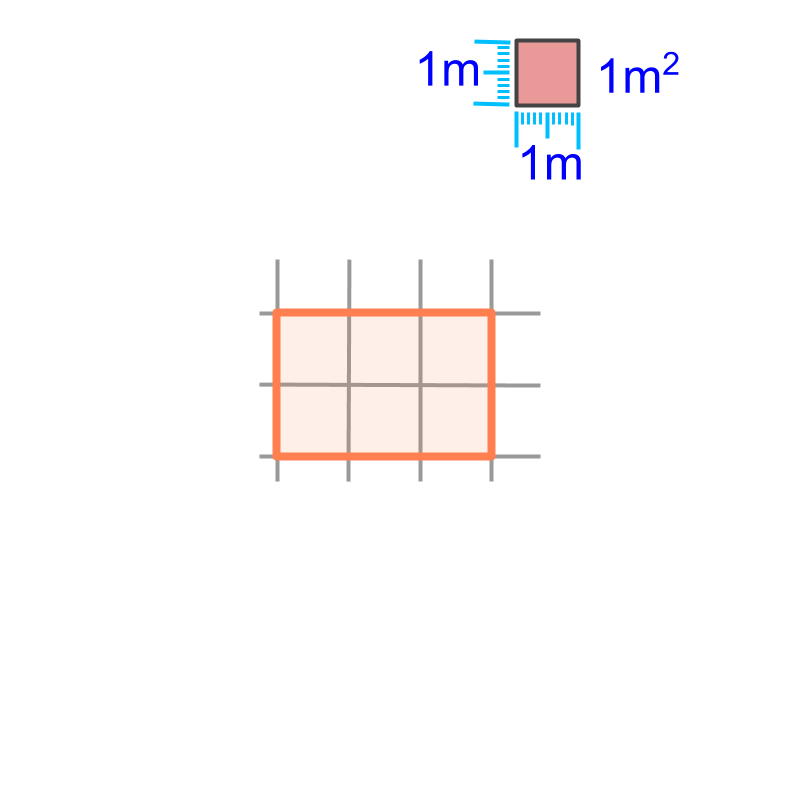•  volume of cubes and cuboids : The unit-cube has ${90}^{\circ }$${90}^{\circ}$ angles at the vertices. And so, the unit-cube fits within cubes and cuboids.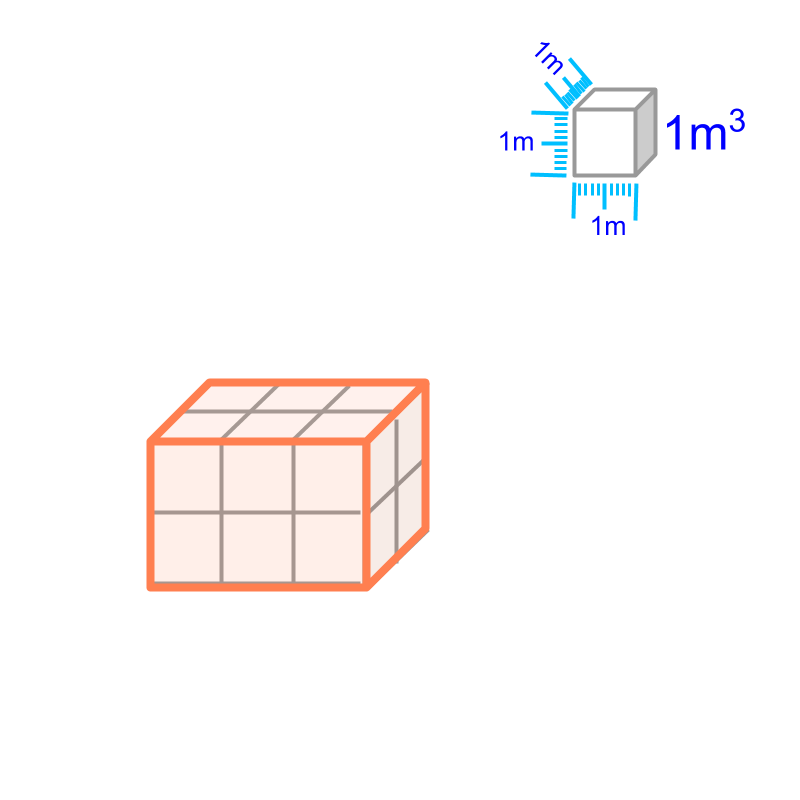For shapes like triangles or prisms, measurement requires some geometrical calculation or the measurement will be approximate.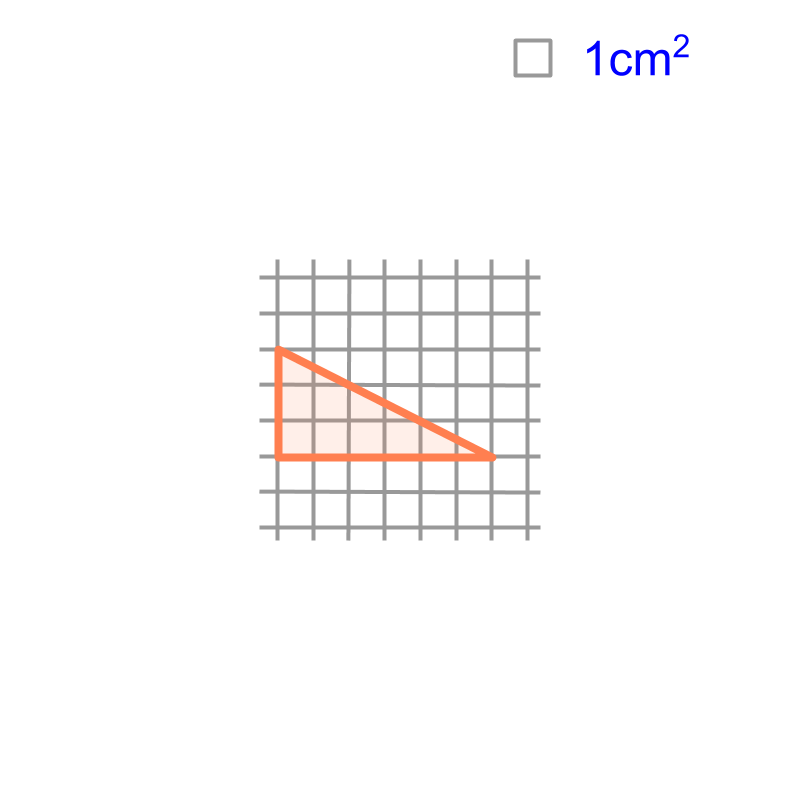summary

Measurement by Superimposition : Length, Area, or Volume can be measured by superimposition of corresponding unit-measures.This method suits best for

•  area of squares and rectangles

•  volume of cubes and cuboids

Outline